# GMAT Math : DSQ: Calculating the ratio of diameter and circumference

## Example Questions

### Example Question #1 : Dsq: Calculating The Ratio Of Diameter And Circumference

Find circle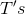ratio of diameter to circumference.

I) The area of circle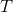is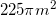.

II) Chord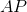passes withinmeter of the center and has a length ofmeters.

Statement II is sufficient to answer the question, but statement I is not sufficient to answer the question.

Both statements are needed to answer the question.

Either statement is sufficient to answer the question.

Statement I is sufficient to answer the question, but statement II is not sufficient to answer the question.

Either statement is sufficient to answer the question.

Explanation:

To find the ratio of diameter to circumference we need our diameter and circumference.

We can use I to find our radius and from there our diameter and circumference.

II can be used to make a triangle with sides of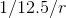which we can then use to find the radius and from there the diameter/circumference.

Either statement is sufficient to answer the question.

### Example Question #2 : Dsq: Calculating The Ratio Of Diameter And Circumference

What is the ratio of the circumference to the diameter of Circle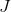?

1.) The radius of the circle is.

2.) The area of the circle is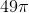Both statements taken together are sufficient to solve the question.

Statement 1 is sufficient to solve the question, but Statement 2 is not sufficient to solve the question.

Each statement alone is sufficient to solve the question.

Neither statement is sufficient to solve the question. More information is needed.

Statement 2 is sufficient to solve the question, but Statement 1 is not sufficient to solve the question.

Each statement alone is sufficient to solve the question.

Explanation:

We are asked to find the ratio of the circumferenceto the diameterof Circleand are given the radius and the area. We also know that.  Taking each statement individually:

1.) The radiusisand we know that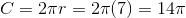. The diameter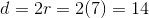. Since we can determine that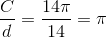,  Statement 1 is sufficient to solve for the ratio by itself.

2.) The areaof the outside circle is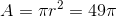, so therefore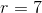. Since we can useto determine both the circumferenceand diameter, Statement 2 is sufficient to solve for the ratio by itself.

### Example Question #3 : Dsq: Calculating The Ratio Of Diameter And Circumference

Circle A and circle B are given. If the diameter of circle B is, what is the diameter of circle A

1. The circumference of circle A is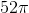.
2. The ratio of the diameters of circle A and circle B is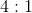, respectively.

Both statements taken together are sufficient to answer the question, but neither statement alone is sufficient.

Each statement alone is sufficient to answer the question.

Statement 1 alone is sufficient, but statement 2 alone is not sufficient to answer the question.

Statement 2 alone is sufficient, but statement 1 alone is not sufficient to answer the question.

Statements 1 and 2 are not sufficient, and additional data is needed to answer the question.

Each statement alone is sufficient to answer the question.

Explanation:

Statement 1: If we know the circumference, we can calculate the diameter.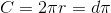If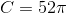then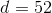Statement 2: We know the diameter of circle B isand that the ratio of the circles. We can set up our proportions and find the diameter: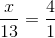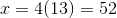### Example Question #4 : Dsq: Calculating The Ratio Of Diameter And Circumference

What is the diameter of the circle?

1. The diameter to radius ratio is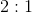.
2. The circumference is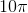.

Statements 1 and 2 are not sufficient, and additional data is needed to answer the question.

Statement 2 alone is sufficient, but statement 1 alone is not sufficient to answer the question.

Statement 1 alone is sufficient, but statement 2 alone is not sufficient to answer the question.

Both statements taken together are sufficient to answer the question, but neither statement alone is sufficient.

Each statement alone is sufficient to answer the question.

Statement 2 alone is sufficient, but statement 1 alone is not sufficient to answer the question.

Explanation:

Statement 1: We're given a ratio (which you should already know) but no values. We need additional information to answer the question.

Statement 2: If we're given the circumference, we can solve for the diameter.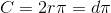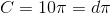which means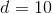Statement 2 alone is sufficient, but statement 1 alone is not sufficient to answer the question.

### Example Question #5 : Dsq: Calculating The Ratio Of Diameter And Circumference

What is the circumference of the circle?

1. The diameter of the circle is.
2. The area of the circle is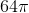.

Each statement alone is sufficient to answer the question.

Statement 1 alone is sufficient, but statement 2 alone is not sufficient to answer the question.

Statements 1 and 2 are not sufficient, and additional data is needed to answer the question.

Statement 2 alone is sufficient, but statement 1 alone is not sufficient to answer the question.

Both statements taken together are sufficient to answer the question, but neither statement alone is sufficient.

Each statement alone is sufficient to answer the question.

Explanation:

Statement 1: We can calculate the circumference using the given diameter.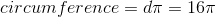Statement 2: To find the circumference, we must first find the radius of the circle using the given area.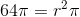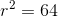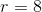We can plug this value into the equation for circumference: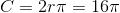Each statement alone is sufficient to answer the question.

Tired of practice problems?

Try live online GMAT prep today.